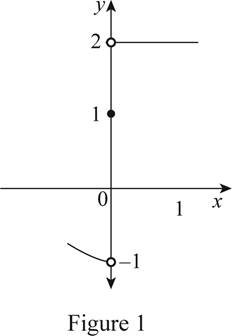# The graph of f ( x ) which satisfies the conditions, lim x → 0 − f ( x ) = − 1 , lim x → 0 + f ( x ) = 2 and f ( 0 ) = 1 .### Single Variable Calculus: Concepts...

4th Edition
James Stewart
Publisher: Cengage Learning
ISBN: 9781337687805### Single Variable Calculus: Concepts...

4th Edition
James Stewart
Publisher: Cengage Learning
ISBN: 9781337687805

#### Solutions

Chapter 2.2, Problem 13E
To determine

## To sketch: The graph of f(x) which satisfies the conditions, limx→0−f(x)=−1,limx→0+f(x)=2 and f(0)=1.

Expert Solution

### Explanation of Solution

Graph:

Given that, limx0f(x)=1,limx0+f(x)=2 and f(0)=1.

Here, the left hand limit and the right hand limit of limx0f(x) are not the same. So, plot the open dot on (0, 2) and (0, −1).

Moreover, the graph must contain a point (0, 1) as f(0)=1. So, mark the point (0, 1) as a filled dot.

Thus, the possible graph of f(x) is shown below in Figure 1.In Figure 1, open dots indicate that the points are excluded.

### Have a homework question?

Subscribe to bartleby learn! Ask subject matter experts 30 homework questions each month. Plus, you’ll have access to millions of step-by-step textbook answers!